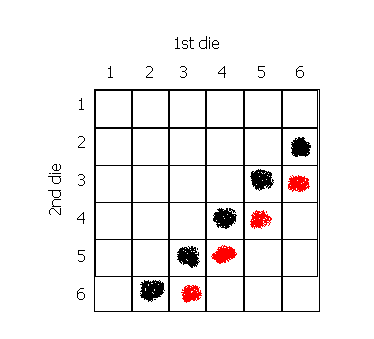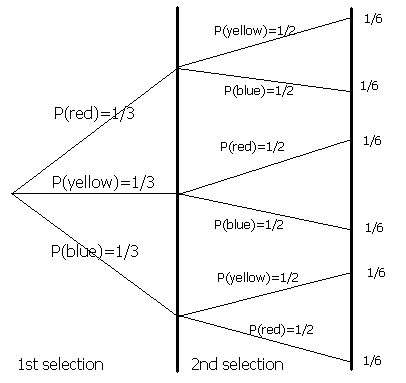## Probability

Introduction

Probability is the likelihood or chance of an event occurring.

 Probability = the number of ways of achieving success the total number of possible outcomes

For example, the probability of flipping a coin and it being heads is ½, because there is 1 way of getting a head and the total number of possible outcomes is 2 (a head or tail). We write P(heads) = ½ .

• The probability of something which is certain to happen is 1.
• The probability of something which is impossible to happen is 0.
• The probability of something not happening is 1 minus the probability that it will happen.

This video is a guide to probability. Expressing probability as fractions and percentages based on the ratio of the number ways an outcome can happen and the total number of outcomes is explained. Experimental probability and the importance of basing this on a large trial is also covered.

Single Events

Example

There are 6 beads in a bag, 3 are red, 2 are yellow and 1 is blue. What is the probability of picking a yellow?

The probability is the number of yellows in the bag divided by the total number of balls, i.e. 2/6 = 1/3.

Example

There is a bag full of coloured balls, red, blue, green and orange. Balls are picked out and replaced. John did this 1000 times and obtained the following results:

• Number of blue balls picked out: 300
• Number of red balls: 200
• Number of green balls: 450
• Number of orange balls: 50

a) What is the probability of picking a green ball?

For every 1000 balls picked out, 450 are green. Therefore P(green) = 450/1000 = 0.45

b) If there are 100 balls in the bag, how many of them are likely to be green?

The experiment suggests that 450 out of 1000 balls are green. Therefore, out of 100 balls, 45 are green (using ratios).

Multiple Events

Independent and Dependent Events

Suppose now we consider the probability of 2 events happening. For example, we might throw 2 dice and consider the probability that both are 6's.

We call two events independent if the outcome of one of the events doesn't affect the outcome of another. For example, if we throw two dice, the probability of getting a 6 on the second die is the same, no matter what we get with the first one- it's still 1/6.

On the other hand, suppose we have a bag containing 2 red and 2 blue balls. If we pick 2 balls out of the bag, the probability that the second is blue depends upon what the colour of the first ball picked was. If the first ball was blue, there will be 1 blue and 2 red balls in the bag when we pick the second ball. So the probability of getting a blue is 1/3. However, if the first ball was red, there will be 1 red and 2 blue balls left so the probability the second ball is blue is 2/3. When the probability of one event depends on another, the events are dependent.

Possibility Spaces

When working out what the probability of two things happening is, a probability/ possibility space can be drawn. For example, if you throw two dice, what is the probability that you will get: a) 8, b) 9, c) either 8 or 9?a) The black blobs indicate the ways of getting 8 (a 2 and a 6, a 3 and a 5, ...). There are 5 different ways. The probability space shows us that when throwing 2 dice, there are 36 different possibilities (36 squares). With 5 of these possibilities, you will get 8. Therefore P(8) = 5/36 .
b) The red blobs indicate the ways of getting 9. There are four ways, therefore P(9) = 4/36 = 1/9.
c) You will get an 8 or 9 in any of the 'blobbed' squares. There are 9 altogether, so P(8 or 9) = 9/36 = 1/4 .

Probability Trees

Another way of representing 2 or more events is on a probability tree.

Example

There are 3 balls in a bag: red, yellow and blue. One ball is picked out, and not replaced, and then another ball is picked out.The first ball can be red, yellow or blue. The probability is 1/3 for each of these. If a red ball is picked out, there will be two balls left, a yellow and blue. The probability the second ball will be yellow is 1/2 and the probability the second ball will be blue is 1/2. The same logic can be applied to the cases of when a yellow or blue ball is picked out first.

In this example, the question states that the ball is not replaced. If it was, the probability of picking a red ball (etc.) the second time will be the same as the first (i.e. 1/3).

This video shows examples of using probability trees to work out the overall probability of a series of events are shown. Both independent and conditional probability are covered.

The AND and OR rules (HIGHER TIER)

In the above example, the probability of picking a red first is 1/3 and a yellow second is 1/2. The probability that a red AND then a yellow will be picked is 1/3 × 1/2 = 1/6 (this is shown at the end of the branch). The rule is:

• If two events A and B are independent (this means that one event does not depend on the other), then the probability of both A and B occurring is found by multiplying the probability of A occurring by the probability of B occurring.

The probability of picking a red OR yellow first is 1/3 + 1/3 = 2/3. The rule is:

• If we have two events A and B and it isn't possible for both events to occur, then the probability of A or B occuring is the probability of A occurring + the probability of B occurring.

On a probability tree, when moving from left to right we multiply and when moving down we add.

Example

What is the probability of getting a yellow and a red in any order?
This is the same as: what is the probability of getting a yellow AND a red OR a red AND a yellow.
P(yellow and red) = 1/3 × 1/2 = 1/6
P(red and yellow) = 1/3 × 1/2 = 1/6
P(yellow and red or red and yellow) = 1/6 + 1/6 = 1/3